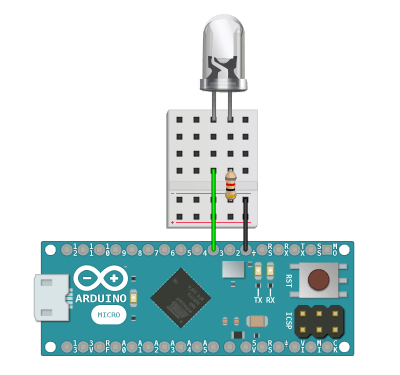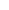Arduino

## LightAPI DOCUMENTATION

Control many lights.

Overview

Control many lights and blink without delay using commands such as on()off()blink(), and fade(). Lights can be connected to PWM or Digital Arduino pins to work with this library.

PWM pins are necessary to enable all library features. Digital pins are supported by this library with special conditions documented in setBrightness() and fade().

Example WiringExample SketchArduinoC++
Public Methods

You can use this library without calling update() and most methods will function correctly. You must continuously call update() inside of loop() in order to use theReal-Time methods tagged below.

#### ```RBD::Light ```

RBD::Light constructor(pin)

Create a new light and pass in the PWM or Digital Arduino pin number.

##### EXAMPLE SKETCH
```RBD::Light light(3); // PWM pin 3

void setup() {
...
}
```
light
.on()

Turn on the light.

##### EXAMPLE SKETCH
```void loop() {
light.on();
}
```
light
.off()

Turn off the light.

##### EXAMPLE SKETCH
```void loop() {
light.off();
}
```
light
.setBrightness(value)

PWM pins: pass in an integer between 0 - 255 to set the raw brightness of the light.

Digital pins: pass in an integer less than or equal to 127 to turn off the light. Pass in an integer greater than or equal to 128 to turn on the light.

##### EXAMPLE SKETCH
```void loop() {
}
```
light
.setBrightnessPercent(value)

Pass in an integer between 0 - 100 to set the percentage brightness of the light. This is the same as setBrightness() except with a smaller input domain.

##### EXAMPLE SKETCH
```void loop() {
light.setBrightnessPercent(75); // 75% brightness
}
```
light
.isOn()

Returns true if the current light brightness is at 100%.

##### EXAMPLE SKETCH
```void loop() {
if(light.isOn()) {
...
}
}
```
light
.isOff()

Returns true if the current light brightness is at 0%.

##### EXAMPLE SKETCH
```void loop() {
if(light.isOff()) {
...
}
}
```
light
.getBrightness()

Returns an integer from 0 - 255 of the current light raw brightness.

##### EXAMPLE SKETCH
```void loop() {
light.getBrightness();
}
```
light
.getBrightnessPercent()

Returns an integer from 0 - 100 of the current light percentage brightness. This is the same as getBrightness() except with a smaller output range.

##### EXAMPLE SKETCH
```void loop() {
light.getBrightnessPercent();
}
```
light
.update()Keep calculating brightness values over time. This must be called continuously within loop() in order to blink() or fade() the light.

##### EXAMPLE SKETCH
```void loop() {
light.update();
}
```
light

Pass in unsigned longs for the on time and off time values in milliseconds. Pass in an integer for the last parameter for the number of times the light should blink, which is [optional] and can be left out to make the light repeat the blink cycle forever.

• Light turns ON for the on_time
• Then turns OFF for the off_time
• Optionally repeats this cycle for the number of [times]
##### EXAMPLE SKETCH
```void setup() {
}

void loop() {
light.update();
}
```
light

PWM pins: need unsigned longs passed in for the up, on, down, and off time values in milliseconds. Pass in an integer for the last parameter for the number of times the light should fade, which is [optional] and can be left out to make the light repeat the fade cycle forever.

Digital pins: behave the same as PWM pins except they will blink the light (when increasing past 50% brightness) instead of appearing as a linear fade.

• Light performs a linear fade UP according to the up_time
• Stays ON for the on_time
• Linear fades DOWN over the course of the down_time
• Stays OFF for the off_time
• Optionally repeats this cycle for the number of [times]
##### EXAMPLE SKETCH
```void setup() {